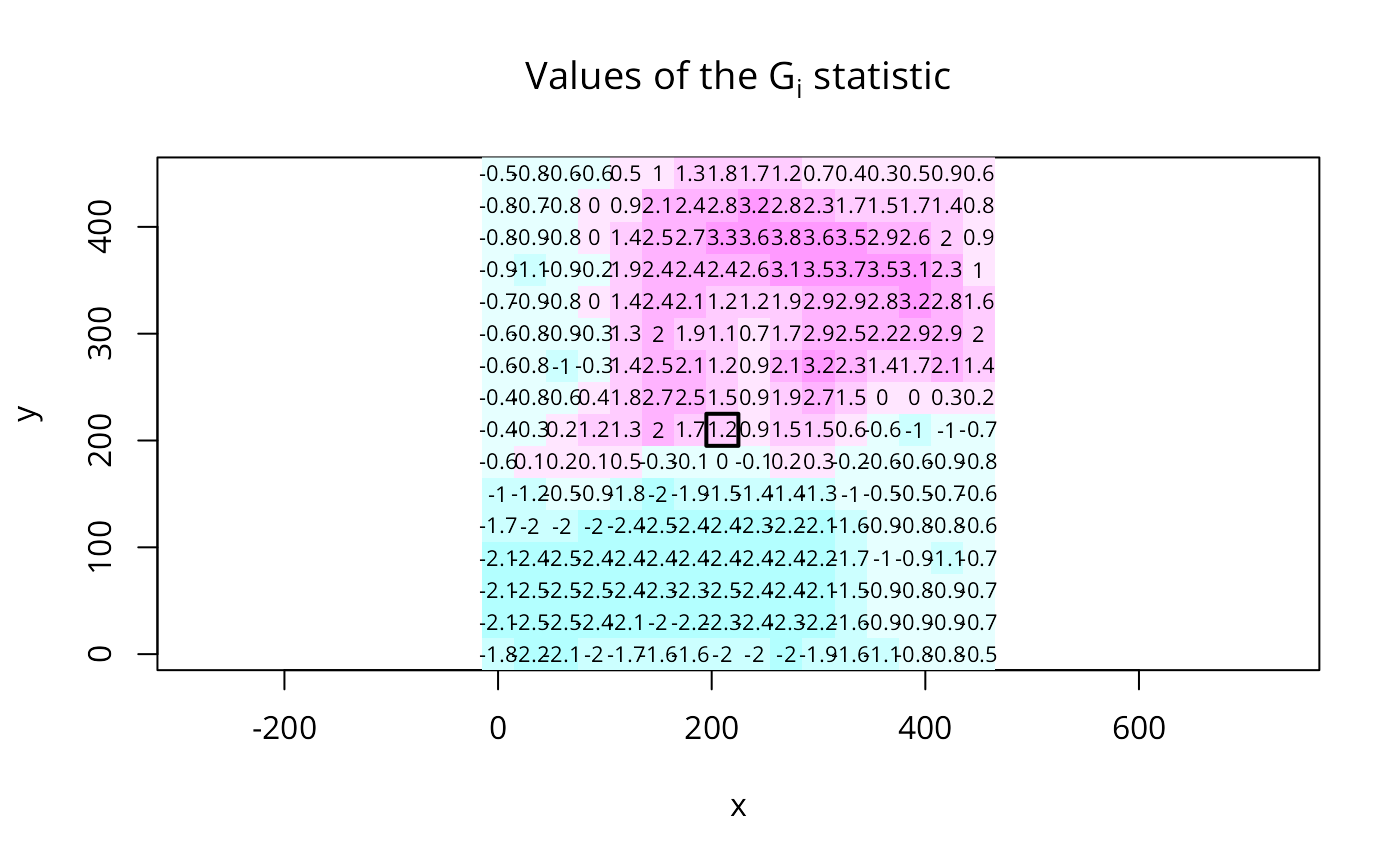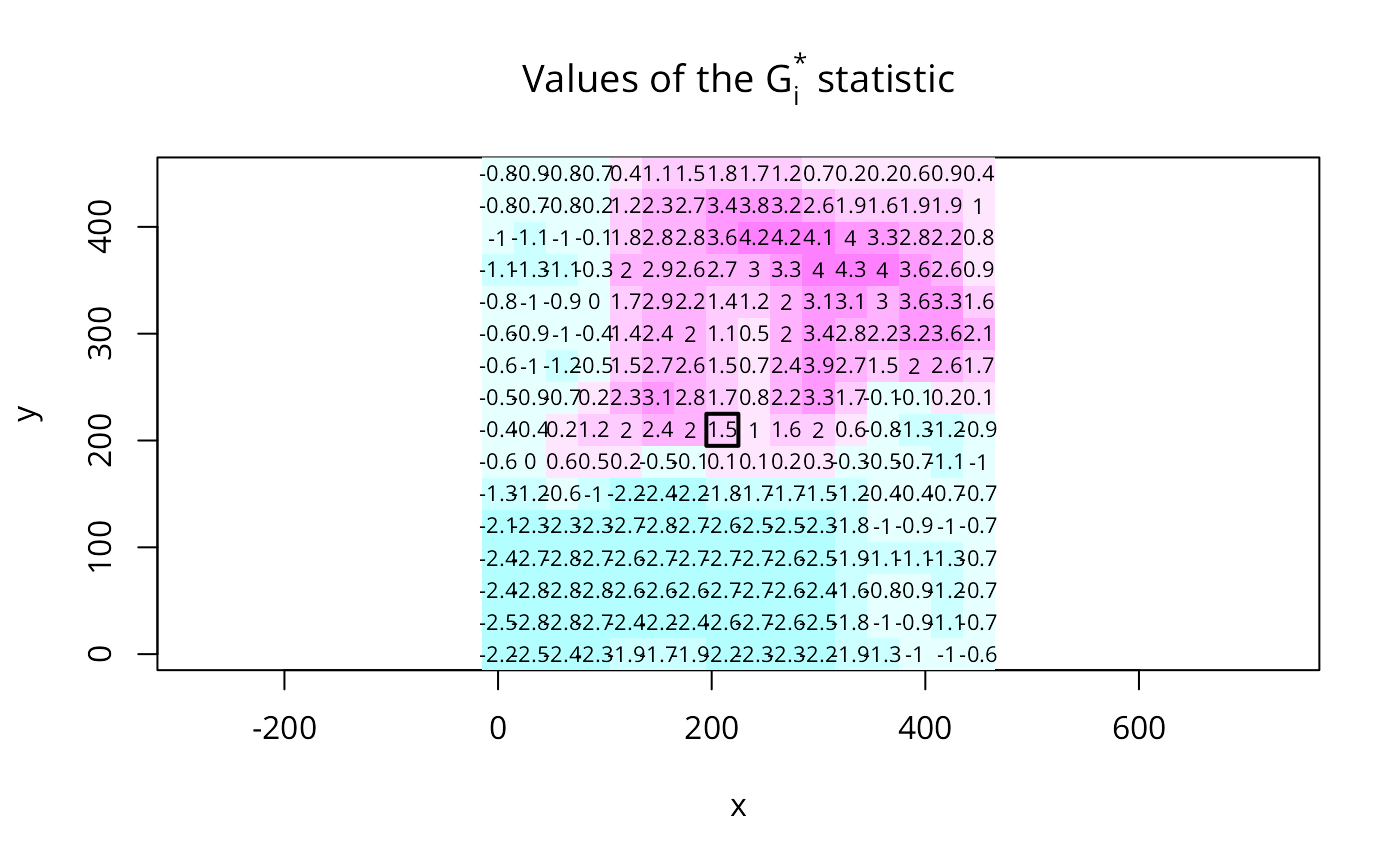The local spatial statistic G is calculated for each zone based on the spatial weights object used. The value returned is a Z-value, and may be used as a diagnostic tool. High positive values indicate the posibility of a local cluster of high values of the variable being analysed, very low relative values a similar cluster of low values. For inference, a Bonferroni-type test is suggested in the references, where tables of critical values may be found (see also details below).

localG(x, listw, zero.policy=NULL, spChk=NULL, GeoDa=FALSE, alternative = "two.sided",
return_internals=TRUE)
localG_perm(x, listw, nsim=499, zero.policy=NULL, spChk=NULL,
alternative = "two.sided", iseed=NULL, fix_i_in_Gstar_permutations=TRUE,
no_repeat_in_row=FALSE)

## Arguments

x

a numeric vector the same length as the neighbours list in listw

listw

a listw object created for example by nb2listw

zero.policy

default NULL, use global option value; if TRUE assign zero to the lagged value of zones without neighbours, if FALSE assign NA

spChk

should the data vector names be checked against the spatial objects for identity integrity, TRUE, or FALSE, default NULL to use get.spChkOption()

GeoDa

default FALSE, if TRUE, drop x values for no-neighbour and self-neighbour only observations from all summations

nsim

default 499, number of conditonal permutation simulations

alternative

a character string specifying the alternative hypothesis, must be one of "two.sided" (default), "greater" or "less".

return_internals

default TRUE, unused

iseed

default NULL, used to set the seed for possible parallel RNGs

fix_i_in_Gstar_permutations

default TRUE (fix x at self in permutations for local G-star), set FALSE to use pre-1.2-8 behaviour

no_repeat_in_row

default FALSE, if TRUE, sample conditionally in each row without replacements to avoid duplicate values, https://github.com/r-spatial/spdep/issues/124

## Details

If the neighbours member of listw has a "self.included" attribute set to TRUE, the Gstar variant, including the self-weight $$w_{ii} > 0$$, is calculated and returned. The returned vector will have a "gstari" attribute set to TRUE. Self-weights must be included by using the include.self function before converting the neighbour list to a spatial weights list with nb2listw as shown below in the example.

The critical values of the statistic under assumptions given in the references for the 95th percentile are for n=1: 1.645, n=50: 3.083, n=100: 3.289, n=1000: 3.886.

## Value

A vector of G or Gstar standard deviate values, with attributes "gstari" set to TRUE or FALSE, "call" set to the function call, and class "localG". For conditional permutation, the returned value is the same as for localG(), and the simulated standard deviate is returned as column "StdDev.Gi" in attr(., "internals").

## Note

Conditional permutations added for comparative purposes; permutations are over the whole data vector omitting the observation itself, and from 1.2-8 fixing the observation itself as its own neighbour for local G-star.

## Author

Roger Bivand Roger.Bivand@nhh.no

## Examples

data(getisord, package="spData")
# spData 0.3.2 changes x, y, xyz object names to go_x, go_y, go_xyz to
# avoid putting these objects into the global environment via lazy loading
if (exists("go_xyz") && packageVersion("spData") >= "0.3.2") {
xyz <- go_xyz
x <- go_x
y <- go_y
}
xycoords <- cbind(xyz$x, xyz$y)
nb30 <- dnearneigh(xycoords, 0, 30)
G30 <- localG(xyz$val, nb2listw(nb30, style="B")) G30[length(xyz$val)-136]
#>  1.221979
set.seed(1)
G30_sim <- localG_perm(xyz$val, nb2listw(nb30, style="B")) G30_sim[length(xyz$val)-136]
#>  1.221979
nb60 <- dnearneigh(xycoords, 0, 60)
G60 <- localG(xyz$val, nb2listw(nb60, style="B")) G60[length(xyz$val)-136]
#>  1.748098
nb90 <- dnearneigh(xycoords, 0, 90)
G90 <- localG(xyz$val, nb2listw(nb90, style="B")) G90[length(xyz$val)-136]
#>  1.986135
nb120 <- dnearneigh(xycoords, 0, 120)
G120 <- localG(xyz$val, nb2listw(nb120, style="B")) G120[length(xyz$val)-136]
#>  1.893374
nb150 <- dnearneigh(xycoords, 0, 150)
G150 <- localG(xyz$val, nb2listw(nb150, style="B")) G150[length(xyz$val)-136]
#>  1.237454
brks <- seq(-5,5,1)
cm.col <- cm.colors(length(brks)-1)
image(x, y, t(matrix(G30, nrow=16, ncol=16, byrow=TRUE)),
breaks=brks, col=cm.col, asp=1)
text(xyz$x, xyz$y, round(G30, digits=1), cex=0.7)
polygon(c(195,225,225,195), c(195,195,225,225), lwd=2)
title(main=expression(paste("Values of the ", G[i], " statistic")))G30s <- localG(xyz$val, nb2listw(include.self(nb30), style="B")) cat("value according to Getis and Ord's eq. 14.2, p. 263 (1996)\n") #> value according to Getis and Ord's eq. 14.2, p. 263 (1996) G30s[length(xyz$val)-136]
#>  1.45078
cat(paste("value given by Getis and Ord (1996), p. 267",
"(division by n-1 rather than n \n in variance)\n"))
#> value given by Getis and Ord (1996), p. 267 (division by n-1 rather than n
#>  in variance)
G30s[length(xyz$val)-136] * (sqrt(sum(scale(xyz$val, scale=FALSE)^2)/length(xyz$val)) / sqrt(var(xyz$val)))
#>  1.447943
image(x, y, t(matrix(G30s, nrow=16, ncol=16, byrow=TRUE)),
breaks=brks, col=cm.col, asp=1)
text(xyz$x, xyz$y, round(G30s, digits=1), cex=0.7)
polygon(c(195,225,225,195), c(195,195,225,225), lwd=2)
title(main=expression(paste("Values of the ", G[i]^"*", " statistic")))